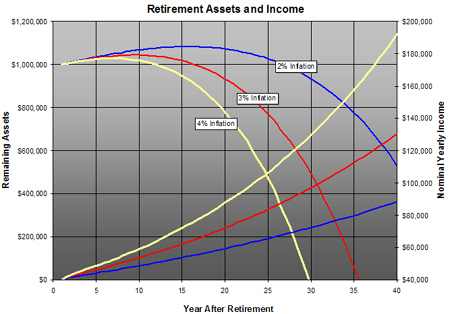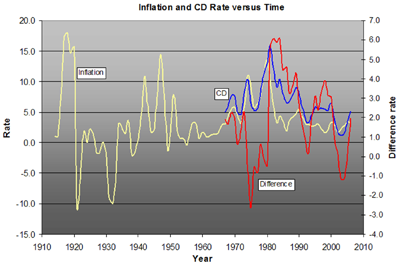## How Long Will Retirement Assets Last?

It is a symptom of middle age that one begins to ponder the prospects of retirement and an occupational affliction of a scientist to marshal mathematics to consider this question. It is difficult to estimate how much income one needs upon retirement. There are many mitigating factors. A retired person is no longer contributing to Social Security and a retirement plan and so this portion of gross income can be dispensed with. The costs of commuting are no longer a factor and usually by the time of retirement one’s house has been paid for and children have left the home. On the other hand, idleness is not a hopeful retirement prospect. Engaging in activities such as travel introduce additional costs. For someone of modest retirement assets, Social Security will form a larger fraction of post-work income. At higher incomes, Social Security drops to a smaller fraction of the total expected income. For baby boomers, there is also the prospect that demographic trends will place strong downward pressure on Social Security payments, particularly for those whose may have other resources.

Here we put off the question of exactly how much income one needs and try to compute for a given amount of retirement assets, what is a reasonable spending level that will allow those assets to last a lifetime. There are two key factors to answering this question: (1) What is a reasonable rate of return on accumulated assets? (2) How much will inflation erode these assets. To answer this question, we performed a simple simulation, the results of which are shown below in Figure 1.Figure 1. Retirement scenarios.

We begin the simulation with \$1,000,000 in retirement assets. Any values we compute can easily be scaled based on actual assets. We also begin with the assumption that we can safely expect a long-term investment rate of return of 5%. We then assume that the first year we withdraw an income \$40,000 (4% of the total). If we started with \$2,000,000 in retirement assets, the yearly income would scale to \$90,000.For the each subsequent year we increase the withdrawal amount by the inflation rate. Overtime, the \$40,000 withdrawal gets nominally larger to maintain a real income of \$40,000.

The blue curve starting at year 0 shows the remaining assets as a function of time for a 2% inflation rate (left scale). Note that for more than the first 25 years, there is actual growth in the nominal level of assets.

The other blue curve represents the nominal income as a function of time (right scale). After 40 years, the nominal income under this scenario would grow to nearly \$90,000, but it would presumably purchase what \$40,000 would have bought at the start of retirement.Note further, that after 40 years, there are sill significant assets remaining, nominally over \$500,000. Assuming a retirement age of 65, retirement assets would still remain at age 105.

The red and yellow curves represent the results of similar scenarios assuming inflation rates of 3% and 4%, respectively. Even with a 3% inflation rate, we could maintain a \$40,000 real income until age 100. At 4% inflation (or a real rate of 1% for a nominal 5% investment return), funds would last until age 95. In other words, if we can maintain real rates of return on our investment of 1% or greater, a retirement income of 4% of the original assets should should last a lifetime.

How reasonable is it to assume that such rates could last over the three or four decades of retirement? As Physicist Neils Bohr once quipped, “Prediction is very difficult, especially about the future.” If we knew with certainty future inflation rates, investment rates of return, and the number of years we would live, retirement planning would be substantially easier. We can, however, look at the past to determine whether our assumptions about the future are plausible.Figure 2. Comparison of CPI-derived inflation rates with CD rates.

The yellow curve in Figure 2 is the inflation rate computed from the Consumer Price Index for Urban Areas (CPI-U) from the Bureau of Labor Statistics from 1913 to 2006 (left scale). There have been many oscillations in the past, yet over the last few decades these extremes seem to have modulated. The end of the 1970’s was the last time that we suffered under double-digit inflation. From the 1980’s to the present we have enjoyed inflation rates less that 5%, usually much less.

For our purposes here, the relationship between inflation rates and the nominal rates of return on safe investments is what matters. As long as the investment rate of return on accumulated assets is appreciably larger than the inflation rate, then inflation risks are alleviated. The blue curve inf Figure 2 represents the rate of return from an average of 3-month Certificate of Deport (left scale). Usually, but not always. CDs have rates of return greater than the inflation rate.The red curve is the difference between the CD and CPI-derived inflation rates (right scale). Since 1968, the period over which we have managed to find data, the difference between the two has averaged 2%. For the scenarios outlined in Figure 1, assuming an investment rate of return of 5% and inflation rate of 3% seems reasonable.

There are a number of factors which will mitigate inflation risks associated with retirement. First, a CD rate of return is about about as conservative as one can get. A slightly more aggressive investment strategy would likely improve the long-term investment rate of return. Moreover, the CPI is thought to overestimate the real inflation rate. We actually might be able to increase withdrawals from assets at a rate lower than the inflation rate and maintain the same standard of living. Finally, any retirement strategy need not be rigid. As the investment returns and inflation change, it is possible to alter the withdrawal strategies as accumulated assets increase or decrease unexpectedly.